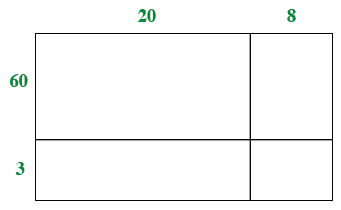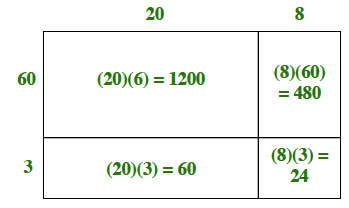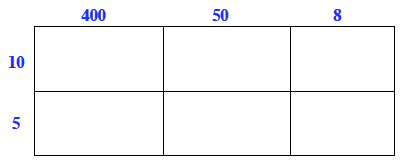### Home > CC1MN > Chapter 3 Unit 3A > Lesson CC1: 3.1.5 > Problem3-77

3-77.

Use the Distributive Property to rewrite each product below. Simplify your answer. Homework Help ✎

1. $28 · 63$

• Write the above product as $(20 + 8)(60 + 3)$ and make a generic rectangle like the one at right.

• Find the product for each part of the rectangle.

• Sum the products: $1200 + 480 + 60 + 24$

• $1764$1. $17(59)$

Write this product as $(10 + 7)(50 + 9)$ and create a generic rectangle similar to the one for part (a).

2. $458(15)$

You can use a generic rectangle like the one below to solve this problem.